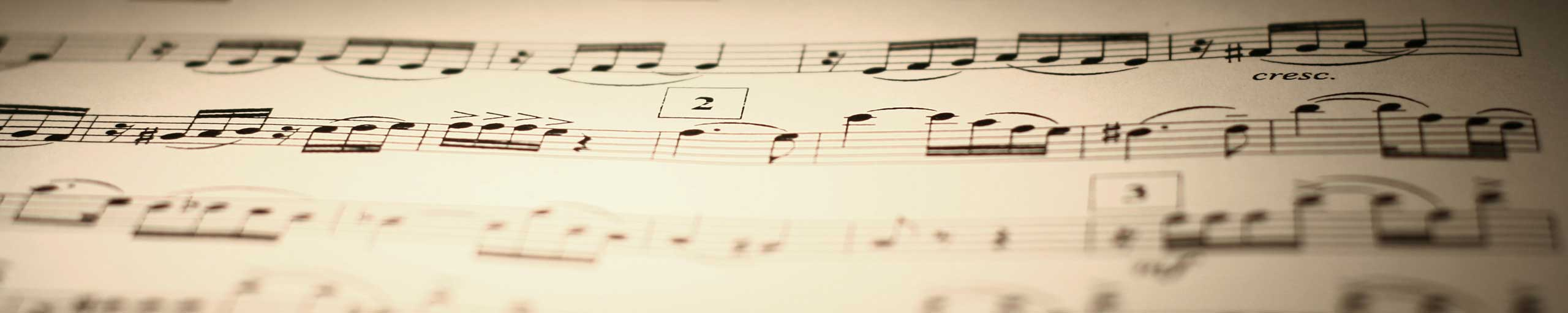# Find the LCM and the GCF of Integers - Examples and Questions with Answers (Grade 5)

How to find the LCM and GCF of integers? Examples and questions with answers. This is a tutorial on how to find the LCM and GCF. In order to help you check your answers, a calculator for the LCM and a calculator for the GFC of two numbers are provided.
Lowest Common Multiple (LCM) Calculator. Calculate the lowest common multiple of two positive integers.
Greatest Common Factor (GCF) Calculator. Calculate the greatest common factor of two positive integers.

## A - Find the LCM of two Numbers

1. GCF = 7 , LCM = 42 , GCF � LCM = 294 , m � n = 21 � 14 = 294
2. GCF = 5 , LCM = 495 , GCF � LCM = 2475 , m � n = 45 � 55 = 2475
3. GCF = 8 , LCM = 3720 , GCF � LCM = 29760 , m � n = 120 � 248 = 29760

More
Primary Math (grades 4 and 5) with Free Questions and Problems With Answers
More
Middle School Math (grades 6,7,8 and 9) with Free Questions and Problems With Answers
More
High School Math (Grades 10, 11 and 12) - Free Questions and Problems With Answers